# KSEEB Solutions for Class 6 Maths Chapter 14 Practical Geometry Ex 14.1

Students can Download Chapter 14 Practical Geometry Ex 14.1 Questions and Answers, Notes Pdf, KSEEB Solutions for Class 6 Maths helps you to revise the complete Karnataka State Board Syllabus and score more marks in your examinations.

## Karnataka State Syllabus Class 6 Maths Chapter 14 Practical Geometry Ex 14.1

Question 1.
Draw a circle of radius 3.2 cm.
Solution: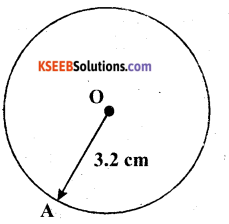The required circle can be drawn as follows.
Step 1: First, open the compasses for the required radius 3.2 cm.
Step 2 : Mark a point ‘O’ where we want the centre of the circle to be.
Step 3 : Place the pointer of compasses on O.
Step 4 : Turn the compasses slowly to draw the circle.Question 2.
With the same centre O, draw two circles of radii 4 cm and 2.5 cm.
Solution: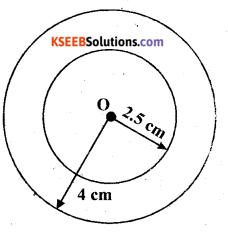The required circle can be drawn as follows.
Step 1 : First, open the compasses for the required radius 4 cm.
Step 2 : Mark a point ‘O’ where we want the centre of the circle to be.
Step 3 : Place the pointer of compasses on O.
Step 4 : Turn the compasses slowly to draw the circle.
Step 5 : Now, open the compasses for 2.5 cm.
Step 6 : Again put the pointer of the compasses on point ‘O’ and turn the compasses slowly to draw the circle.Question 3.
Draw a circle and any two of its diameters. If you join the ends of these diameters, what is the figure obtained? What figure is obtained if the diameters are perpendicular to each other? How do you check your answer?
Solution:
A circle can be drawn of any convenient radius, also having its centre as 0. Let AB and CD be two diameters of this circle. When we join the ends of these diameters, a quadrilateral ACBD is formed.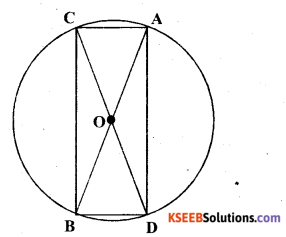As we know that the diameters of a circle are equal in length, therefore, the quadrilateral so formed will have its diagonals of equal length.
Also, OA = OB = OC = OD = radius r and if a quadrilateral has its diagonals of same length which are bisecting each other, then it will be a rectangle.
Let DE and FG be two diameters of this circle such that these are perpendicular to each other. A quadrilateral is formed by joining the ends of these diameters.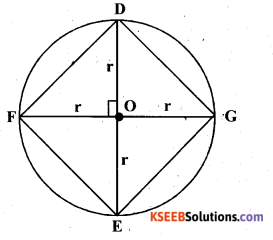Here, OD = OE = OF = OG = radius r
In this quadrilateral DFEQ the diagonals are equal and perpendicular to each other. Also, since these are bisecting each other, it will be a square.
The length of the sides of the quadrilateral so formed can be measured to check our answers.Question 4.
Draw any circle and mark points A, B and C such that
(a) A is on the circle.
(b) B is in the interior of the circle.
(c) C is in the exterior of the circle.
Solution: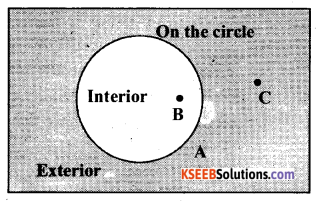Question 5.
Let A, B be the centres of two circles of equal radii; draw them so that each one of them passes through the centre of the other. Let them intersect at C and D. Examine whether $$\overline{\mathbf{A B}}$$ and $$\overline{\mathbf{C D}}$$ are at right angles.
Solution:
Let us draw two circles of same radius which are passing through the centres of the other circle.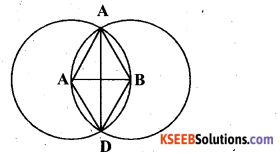Here, point A and B are the centres of these circles and these circles are intersecting each other at point C and D.
Hence, $$\overline{\mathbf{A B}}$$ and $$\overline{\mathbf{C D}}$$ are at right angles.